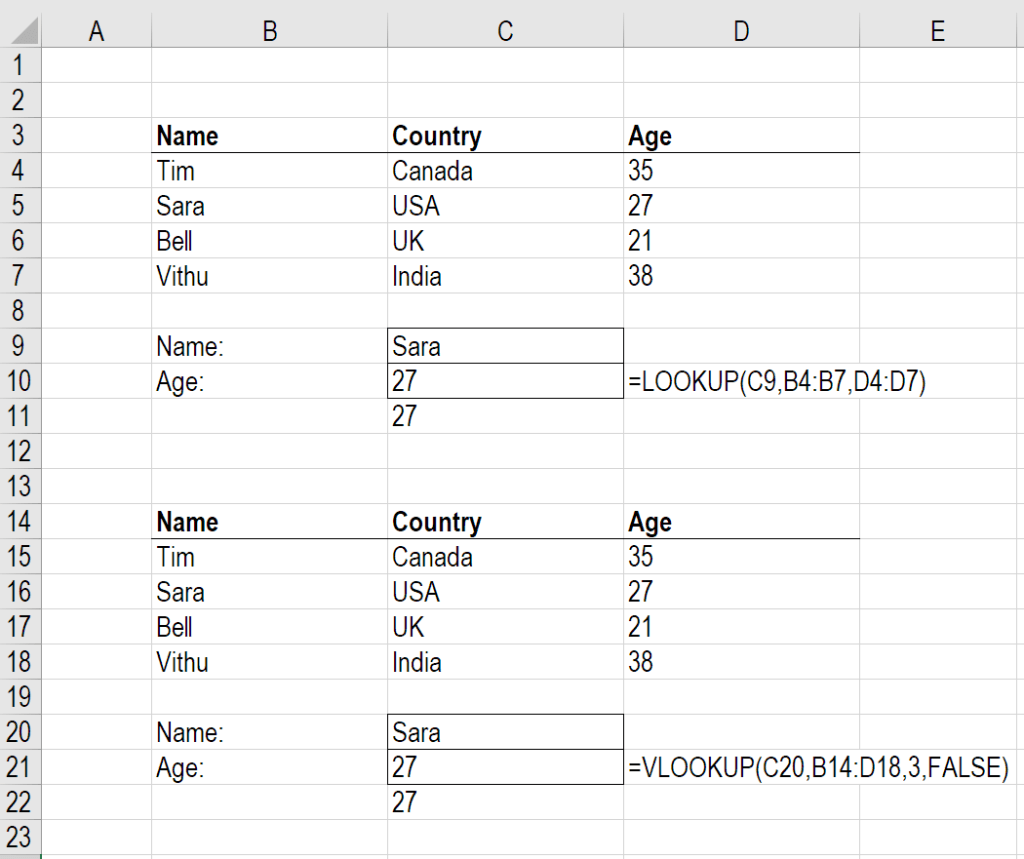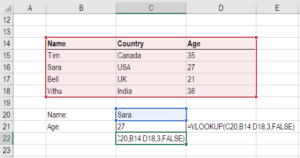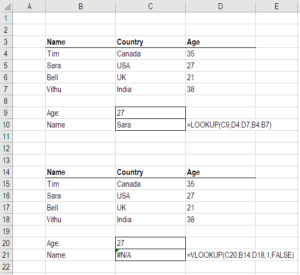# LOOKUP vs VLOOKUP

Comparing the LOOKUP and VLOOKUP Functions in Excel

## What are the LOOKUP vs VLOOKUP Functions?

Knowing the difference between LOOKUP vs VLOOKUP Functions in Excel enables users to take full advantage of the benefits of using each function. The LOOKUP function allows a user to search for a piece of data in a row or column and return a corresponding piece of data in another row or column. The VLOOKUP function is similar but only allows a user to search vertically in a row and only returns data in a left-to-right procedure. This guide will outline why it’s better to use LOOKUP instead of VLOOKUP or HLOOKUP in Excel modeling.

### Why use LOOKUP vs VLOOKUP or HLOOKUP?

Simply put, the LOOKUP Function is better than VLOOKUP, as it’s less restrictive in its use.  It was only introduced by Microsoft in 2016, so it’s still new to most users.

Benefits of LOOKUP vs VLOOKUP:

• Users can search for data both vertically (columns) and horizontally (rows)
• Allows for left-to-right and right-to-left procedures (VLOOKUP is only left-to-right)
• Simpler to use and doesn’t require selecting the entire table
• Can replace both VLOOKUP and HLOOKUP functions
• Easier to audit with F2 than VLOOKUP or HLOOKUP

### Examples of LOOKUP vs VLOOKUP

Below are two screenshots of the LOOKUP vs VLOOKUP functions being used in Excel.  As you will see in both examples, there is a simple table with some people’s names, countries, and ages. The tool that’s been built allows the user to enter a person’s name and get Excel to return their age.

##### Example 1: LOOKUP

In this example, the LOOKUP Function is used with the following steps:

1. Select the name that’s entered in cell C9.
2. Search for it in column B4:B7.
3. Return the corresponding age in column D4:D7.As you can see, this formula is very intuitive and easy to audit.

##### Example 2: VLOOKUP

In this second example, VLOOKUP is used with the following steps:

1. Select the name that’s entered in cell C20.
2. Select the range of the entire table B14:D18.
3. Select the corresponding output from column “3.”
4. “False” requires an exact name match.This formula also works, but it’s harder to set up, understand, and audit.

### Right-to-left Functionality

The biggest benefit of LOOKUP vs VLOOKUP may be its ability to operate from right-to-left.  You will see below that if you want to switch the procedure to search for age and output the corresponding name, it works for LOOKUP but produces an error with VLOOKUP.### Index Match Match Alternative

The only limitation of the LOOKUP Function is that it’s not completely dynamic.  If, for example, you wanted to be able to change the output column from Age to Country, you’d need to manually change the formula to refer to a different column.

As a more advanced alternative, you can combine the INDEX and MATCH Functions to automatically switch references.

See CFI’s step by step guide on how to use Index Match Match in Excel.

Thank you for reading CFI’s explanation of the LOOKUP vs VLOOKUP functions. CFI is the official issuer of the Financial Modeling & Valuation Analyst certification program. To continue learning and developing as an analyst, the following free CFI resources will be helpful:

• List of Excel Functions